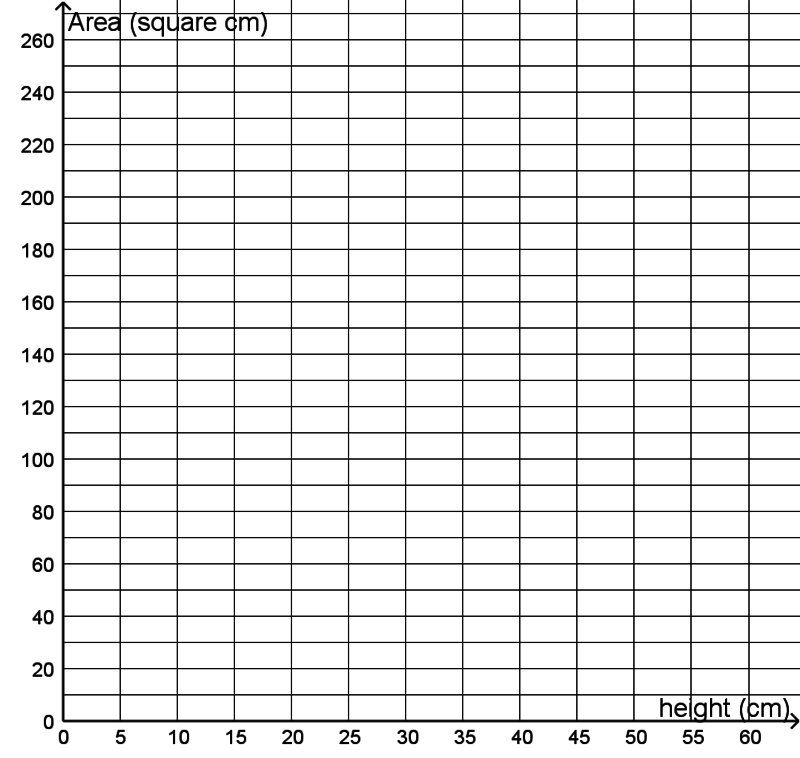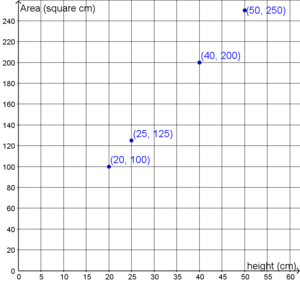# Families of Triangles

Alignments to Content Standards: 6.EE.C.9 6.EE.A.2.c

There are a bunch of triangles. They all have one side that is 10 centimeters long, which we will consider as the base of the triangle.

1. The triangles each have a different height (as measured off of the 10-centimeter base) and so have different areas. Fill in the table:
Height (centimeters) Area (square centimeters)
20
25
40
250
2. Plot the ordered pairs from the table in the coordinate plane and label them with their coordinates.3. Where can you see the answers to part (a) in the coordinate plane?
4. If $A$ represents the area and $h$ represents the corresponding height, write an equation using $A$ and $h$ that represents the area of any such triangle.

## IM Commentary

The purpose of this task is to introduce students to the idea of a relationship between two quantities by using a familiar geometic context. In order to benefit from this task, students should have already developed and become comfortable with a formula for the area of a triangle. The focus of this task should be on noticing the relationship between height and area and creating a graphical and algebraic representation of this relationship, not on understanding the meaning behind the geometric terms.

Even if students have done this work with area, they may need help interpreting the task. For example, the area formula $A=\tfrac{1}{2}bh$ requires one of the sides to be chosen as the "base", relative to which there is a corresponding height.  The task defines the given 10-centimeter-long side to be the base -- a diagram of a triangle where one side is labeled 10 and the corresponding height is drawn and perhaps labeled with a $?$ or with an $h$ might help.

Students should have learned to graph ordered pairs in the first quadrant in grade 5, but since this is a recent skill, teachers should take care to ensure that students are plotting and labeling points correctly. The task doesn't require it, but this task is an opportunity to introduce or reinforce the language of independent variable (in this case height) and dependent variable (in this case area).

In part (d), students engage in MP8 (express regularity in repeated reasoning) when they think about all of the arithmetic they did in part (a) and express that arithmetic using an $h$ in place of a numerical height. This is a skill at the heart of the arithmetic to algebra transition that takes place in grade 6, and many students may find it difficult. One way to scaffold this work might be to ask students to write out in words the arithmetic process they used several times in part (a): "I started with the height, multiplied it by 10, and then divided the result by 2." The work of part (d) is expressing that process using a letter for the height.

## Solution

1. The completed table:

Height (cm) Area (square cm)
20 100
25 125
40 200
50 250
2. The plotted ordered pairs:3. The heights are the $x$-coordinates of the points on the graph and the areas are the $y$-coordinates of the points on the graph.

4. $A=\frac{10h}{2}$, $A=\frac12 \cdot 10h$, $A=5h$, or equivalent.